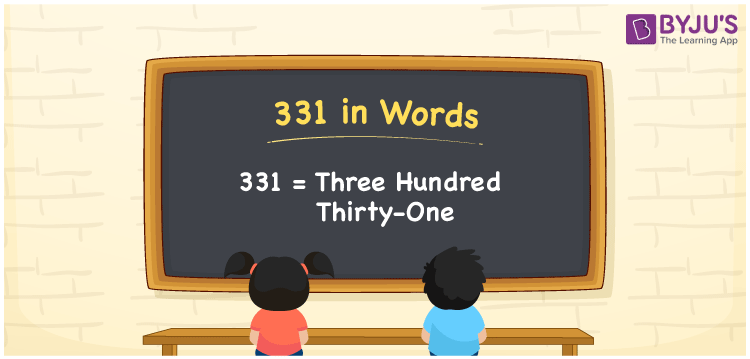# 331 in words

331 in words is written as Three Hundred and Thirty One. 331 represents the count or value. The article on Counting Numbers can give you an idea about count or counting. The number 331 is a 3 digit number that is used in expressions related to money, days, distance, length, weight and so on. Let us consider an example for 331. “We have to wait for Three Hundred and Thirty One days for the new year”.

 331 in words Three Hundred and Thirty One Three Hundred and Thirty One in Numbers 331

## 331 in English Words## How to Write 331 in Words?

We can convert 331 to words using a place value chart. The number 331 has 3 digits, so let’s make a chart that shows the place value up to 3 digits.

 Hundreds Tens Ones 3 3 1

Thus, we can write the expanded form as:

3 × Hundred + 3 × Ten + 1 × One

= 3 × 100 + 3 × 10 + 1 × 1

= 331

= Three Hundred and Thirty One.

331 is the natural number that is succeeded by 330 and preceded by 332.

331 in words – Three Hundred and Thirty One.

Is 331 an odd number? – Yes.

Is 331 an even number? – No.

Is 331 a perfect square number? – No.

Is 331 a perfect cube number? – No.

Is 331 a prime number? – Yes.

Is 331 a composite number? – No.

## Solved Example

1. Write the number 331 in expanded form

Solution: 3 × 100 + 3 × 10 + 1 × 1

We can write 331 = 300 + 30 + 1

= 3 × 100 + 3 × 10 + 1 × 1.

## Frequently Asked Questions on 331 in words

Q1

### How to write the number 331 in words?

331 in words is written as Three Hundred and Thirty One.
Q2

### Is 331 divisible by 3?

No. 331 is not divisible by 3.
Q3

### Is 331 a prime number?

Yes. 331 is a prime number.